Take Profit (Calculate)=Take Profit (Chart)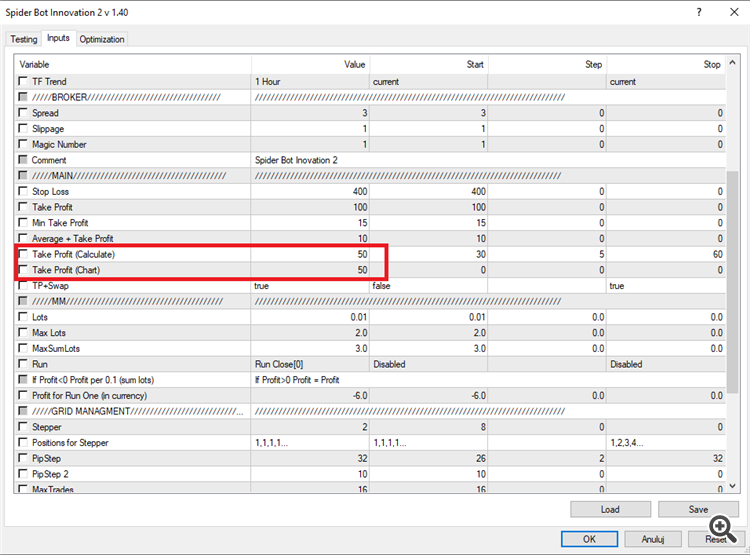Take Profit (Chart)=50 pips on chart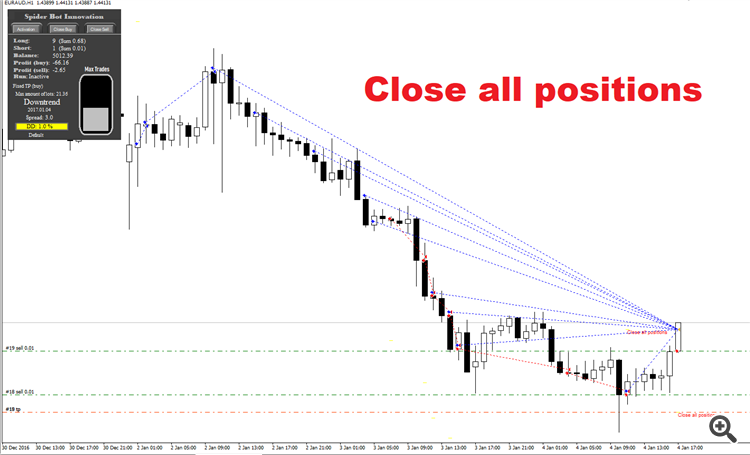Take Profit (Calculate)>Take Profit (Chart)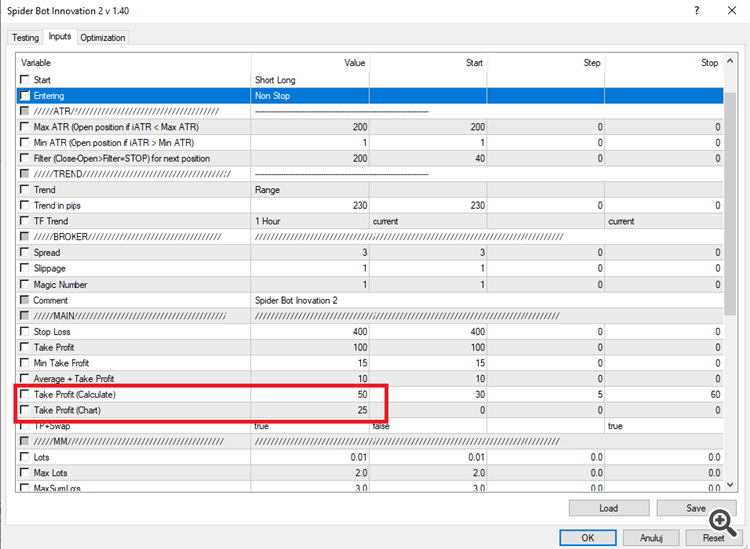Lot size for Take Profit (Calculate) =50 pips

Take Profit (Chart)=25 pips on chart

It shall reduce the net positions by the reduction factors

(Reduction to free up capital through a suitable reduction in risk positions and to enhance efficiency).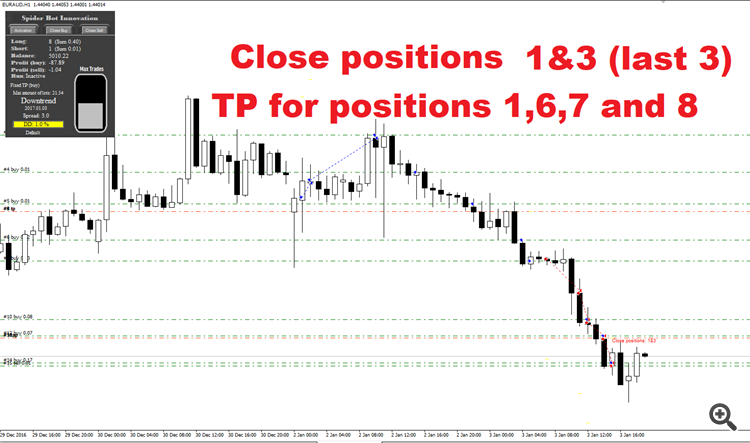Recalculation after closing the position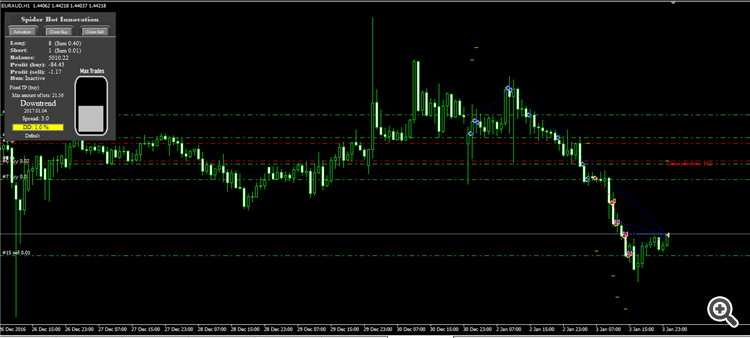Recalculation after opening a new position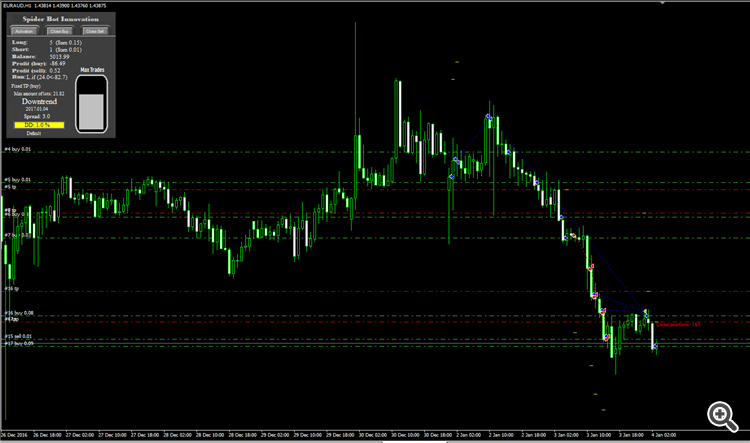Recalculation  after opening a next position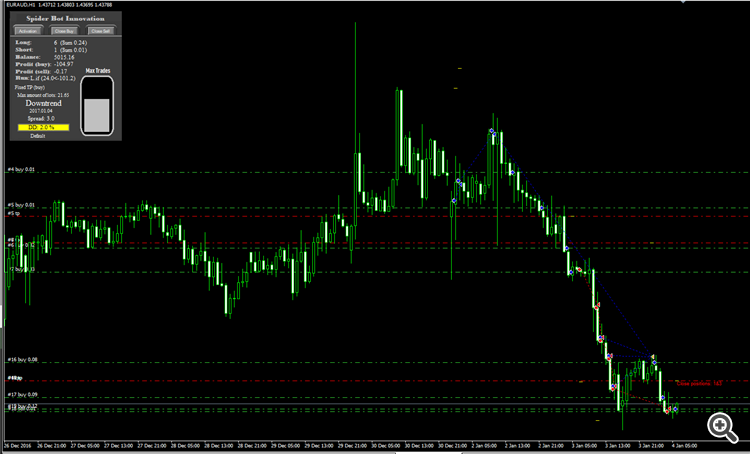e.t.c.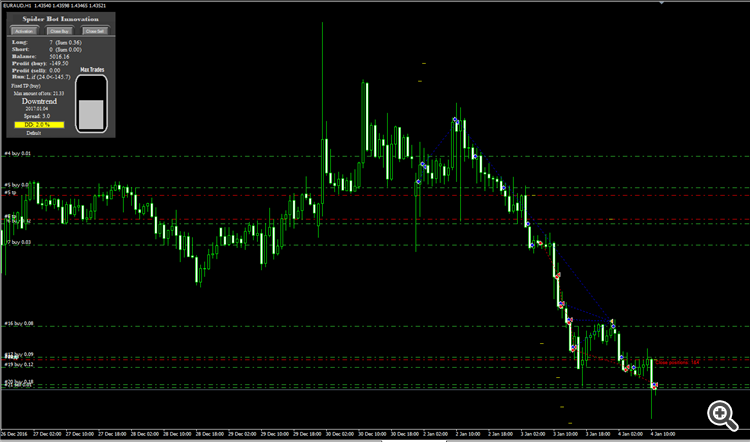e.t.c.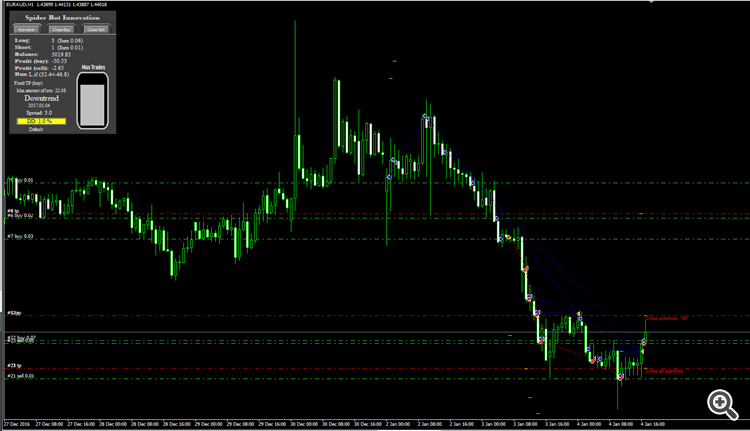Share it with friends: